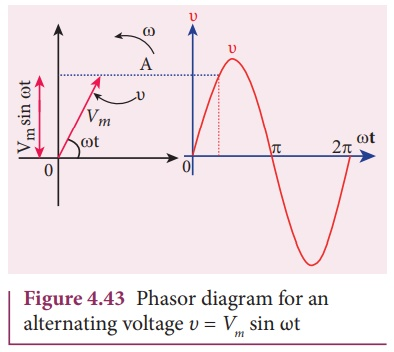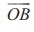Home | | Physics 12th Std | RMS value of AC

# RMS value of AC

The root mean square value of an alternating current is defined as the square root of the mean of the squares of all currents over one cycle.

RMS value of AC

The term RMS refers to time-varying sinusoidal currents and voltages and not used in DC systems.

The root mean square value of an alternating current is defined as the square root of the mean of the squares of all currents over one cycle. It is denoted by IRMS . For alternating voltages, the RMS value is given by VRMS.

The alternating current i = I m sin Žē t or i = Im sin╬Ė ,  is represented graphically in Figure 4.42. The corresponding squared current wave is also shown by the dotted lines.

The sum of the squares of all currents over one cycle is given by the area of one cycle of squared wave. Therefore,An elementary area of thickness d╬Ė is considered in the first half-cycle of the squared current wave as shown in Figure 4.42. Let i2 be the mid-ordinate of the element.

Area of the element = i 2d╬ĖThus we find that for a symmetrical sinusoidal current rms value of current is 70.7 % of its peak value.

Similarly for alternating voltage, it can be shown thatEXAMPLE 4.18

Write down the equation for a sinusoidal voltage of 50 Hz and its peak value is 20 V. Draw the corresponding voltage versus time graph.

SolutionEXAMPLE 4.19

The equation for an alternating current is given by i = 77 sin 314t. Find the peak value, frequency, time period and instantaneous value at t = 2 ms.

Solution

i = 77 sin 314t ; t = 2 ms = 2├Ś10-3 s

The general equation of an alternating current is i = Im sin Žēt . On comparsion,

(i) Peak value, Im = 77 A

(ii) Frequency, f = Žē/2ŽĆ = 314 / 2 ├Ś3.14 = 50 Hz

Time period, T = 1/f = 150 = 0 .02 s

(iv) At t = 2 m s,

Instantaneous value,

i = 77sin(314├Ś2├Ś10ŌłÆ3 )

i = 45.24 A

## Phasor and phasor diagram

### Phasor

A sinusoidal alternating voltage (or current) can be represented by a vector which rotates about the origin in anti-clockwise direction at a constant angular velocity Žē. Such a rotating vector is called a phasor. A phasor is drawn in such a way that

┬Ę        the length of the line segment equals the peak value Vm (or Im) of the alternating voltage (or current)

┬Ę        its angular velocity Žē is equal to the angular frequency of the alternating voltage (or current)

┬Ę        the projection of phasor on any vertical axis gives the instantaneous value of the alternating voltage (or current)

┬Ę        the angle between the phasor and the axis of reference (positive x-axis) indicates the phase of the alternating voltage (or current).

The notion of phasors is introduced to analyse phase relationship between voltage and current in different AC circuits.

### Phasor diagram

The diagram which shows various phasors and their phase relations is called phasor diagram. Consider a sinusoidal alternating voltage Žģ = Vm sin Žēt applied to a circuit. This voltage can be represented by a phasor, namelyas shown in Figure 4.43.Here the length ofequals the peak value (Vm), the angle it makes with x-axis gives the phase (Žēt) of the applied voltage. Its projection on y-axis provides the instantaneous value (Vm sin Žēt) at that instant.Whenrotates about O with angular velocity Žē in anti-clockwise direction, the waveform of the voltage is generated. For one full rotation of, one cycle of voltage is produced.

The alternating current in the same circuit may be given by the relation iŌĆå=ŌĆåImsin (Žēt + ŽĢ) which is represented by another phasor. Here ŽĢ is the phase angle between voltage and current. In this case, the current leads the voltage by phase angle ŽĢ which is shown in Figure 4.44. If the current lags behind the voltage, then we write i = Im sin (Žēt - ŽĢ).

Tags : Phasor and phasor diagram, Definition, Explanation, Formulas, Solved Example Problems | Alternating Current (AC) , 12th Physics : Electromagnetic Induction and Alternating Current
Study Material, Lecturing Notes, Assignment, Reference, Wiki description explanation, brief detail
12th Physics : Electromagnetic Induction and Alternating Current : RMS value of AC | Phasor and phasor diagram, Definition, Explanation, Formulas, Solved Example Problems | Alternating Current (AC)